# Civil Engineering - RCC Structures Design

### Exercise :: RCC Structures Design - Section 5

36.

The minimum thickness of the cover at the end of a reinforcing bar should not be less than twice the diameter of the bar subject to a minimum of

 A. 10 mm B. 15 mm C. 20 mm D. 25 mm E. 30 mm

Explanation:

No answer description available for this question. Let us discuss.

37.

If l1 and l2 are the lengths of long and short spans of a two way slab simply supported on four edges and carrying a load w per unit area, the ratio of the loads split into w1 and w2 acting on strips parallel to l2 and l1 is

 A.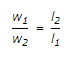B.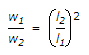C.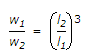D.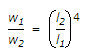Explanation:

No answer description available for this question. Let us discuss.

38.

If L is the effective span of a R.C.C. beam which is subjected to maximum shear qmax at the ends, the distance from either end over which stirrups for the shear, are provided, is

 A.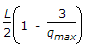B.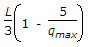C.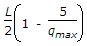D.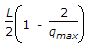Explanation:

No answer description available for this question. Let us discuss.

39.

For the design of a simply supported T-beam the ratio of the effective span to the overall depth of the beam is limited to

 A. 10 B. 15 C. 20 D. 25 E. 30

Explanation:

No answer description available for this question. Let us discuss.

40.

In the zone of R.C.C. beam where shear stress is less than 5 kg/cm2, nominal reinforcement is provided at a pitch of

 A. one-half lever arm of the section B. one-third lever arm of the section C. lever arm of the section D. one and half lever arm of the section.